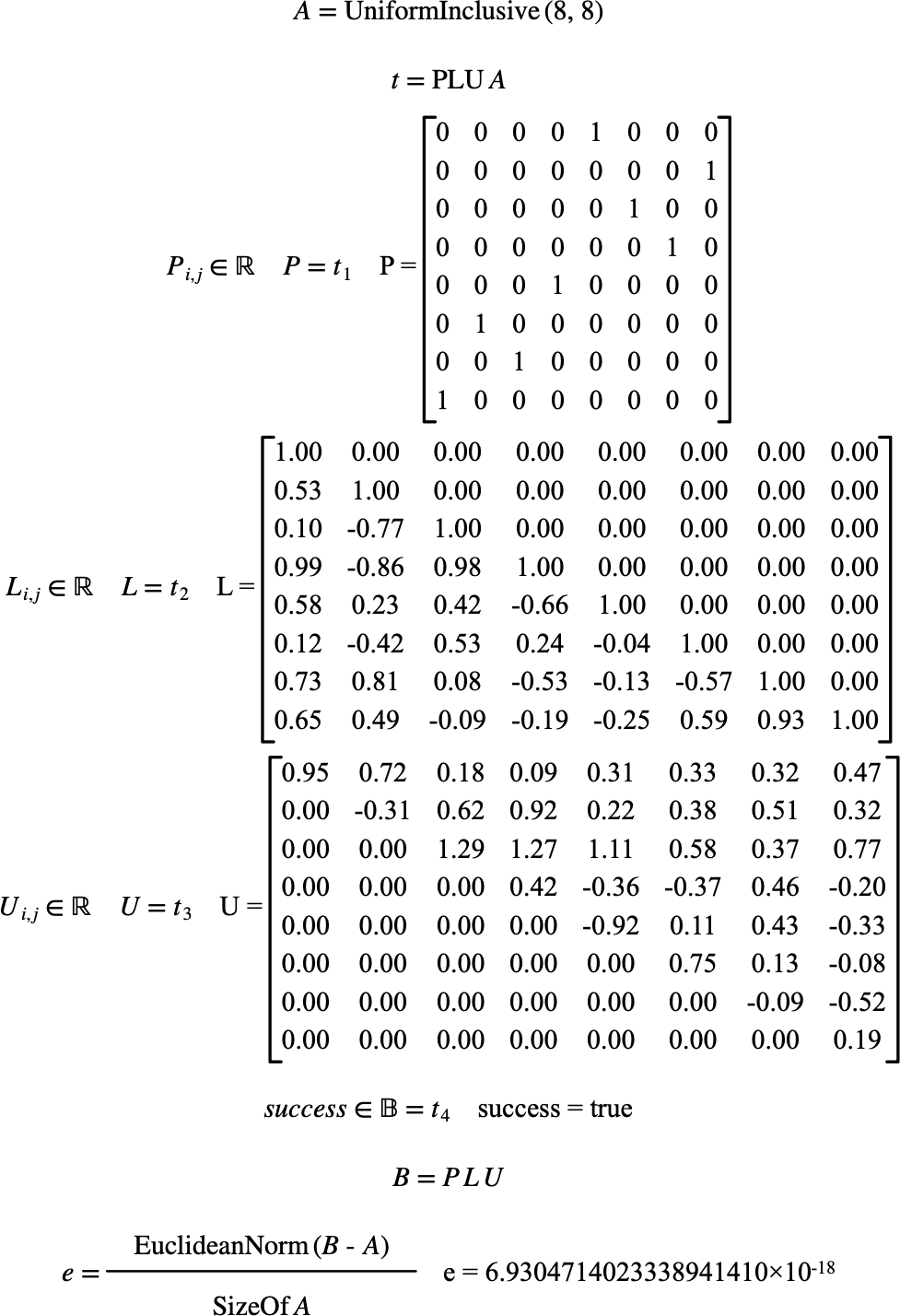# $$\text{PLU}$$¶

You can use the $$\text{PLU}$$ function to perform LU decomposition of a matrix with row pivoting.

You can use the \plu backslash command to insert this function.

The following variants of this function are available:

• $$\text{tuple } \text{PLU} \left ( \text{<matrix>} \right )$$

The $$\text{<matrix>}$$ parameter is the matrix to perform the LU decomposition on.

The $$\text{PLU}$$ function returns a tuple containing:

• A row pivot matrix

• A lower triangular matrix

• An upper triangular matrix

• A boolean flag indicating true on success or false on error.

The function is implemented internally for real and complex matrices although you can supply boolean and integer matrices which will be converted to real matrices prior to performing the decomposition operation.

Figure 182 shows the basic use of the $$\text{PLU}$$ function.Figure 182 Example Use Of The PLU Function